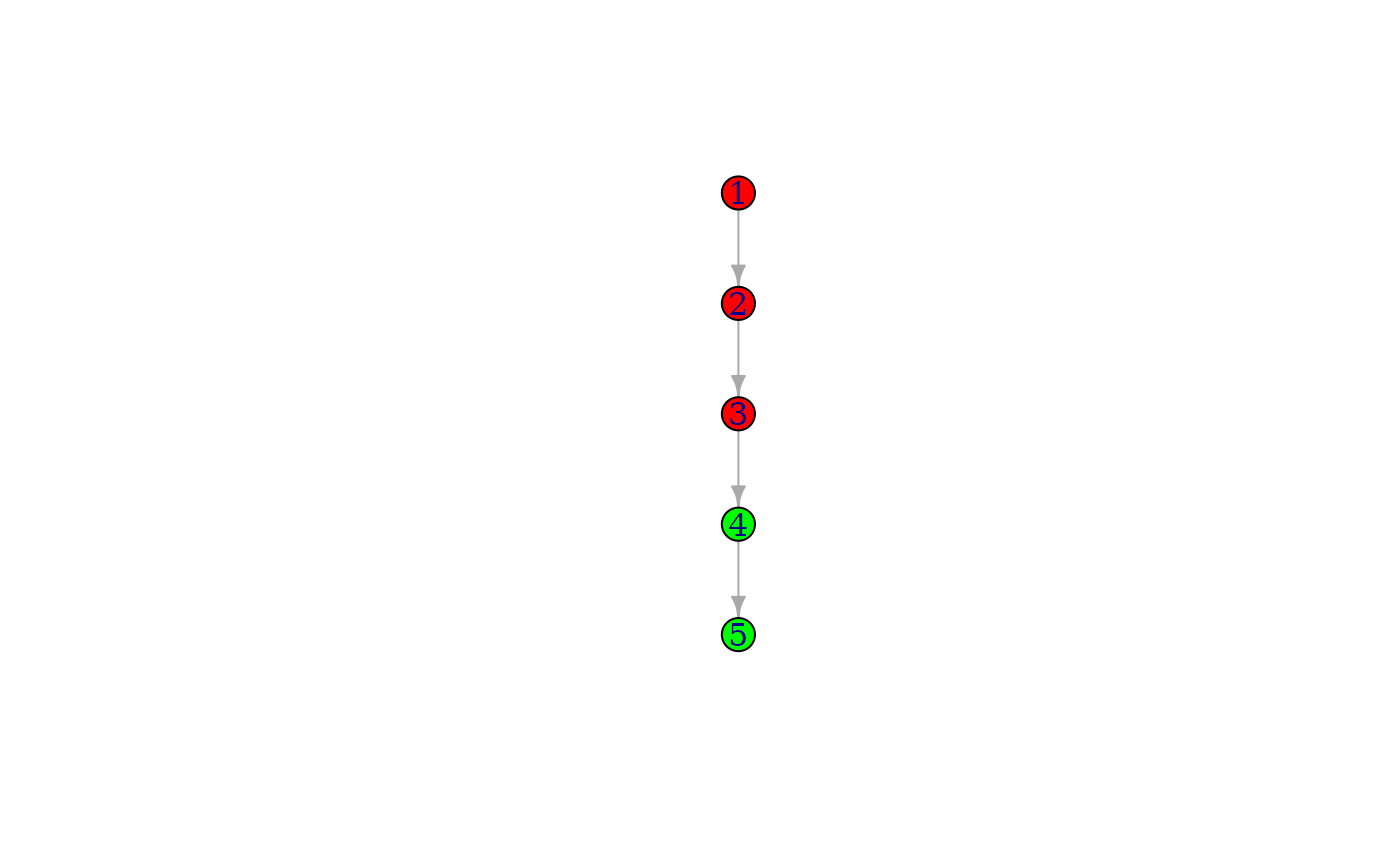If attributes are supplied, and they are not present in the graph, their values for the original vertices of the graph are set to NA.

## Usage

add_vertices(graph, nv, ..., attr = list())

## Arguments

graph

The input graph.

nv

The number of vertices to add.

...

attr

## Value

The graph, with the vertices (and attributes) added.

Other functions for manipulating graph structure: +.igraph(), add_edges(), complementer(), compose(), connect(), contract(), delete_edges(), delete_vertices(), difference.igraph(), difference(), disjoint_union(), edge(), igraph-minus, intersection.igraph(), intersection(), path(), permute(), rep.igraph(), reverse_edges(), simplify(), union.igraph(), union(), vertex()

## Examples

g <- make_empty_graph() %>%
1, 2,
2, 3,
3, 4,
4, 5
))
g
#> IGRAPH 0f6f7cd D--- 5 4 --
#> + attr: color (v/c)
#> + edges from 0f6f7cd:
#>  1->2 2->3 3->4 4->5
V(g)[[]]
#> + 5/5 vertices, from 0f6f7cd:
#>   color
#> 1   red
#> 2   red
#> 3   red
#> 4 green
#> 5 green
plot(g)